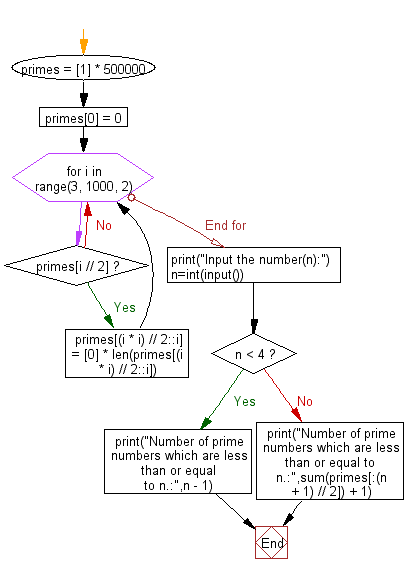﻿ Python: Print the number of prime numbers which are less than or equal to a given integer - w3resource# Python: Print the number of prime numbers which are less than or equal to a given integer

## Python Basic - 1: Exercise-38 with Solution

Write a Python program to print the number of prime numbers which are less than or equal to a given integer.

Input:
n (1 ≤ n ≤ 999,999)
Input the number(n): 35
Number of prime numbers which are less than or equal to n.: 11

Sample Solution:

Python Code:

``````primes =  * 500000
primes = 0

for i in range(3, 1000, 2):
if primes[i // 2]:
primes[(i * i) // 2::i] =  * len(primes[(i * i) // 2::i])

print("Input the number(n):")
n=int(input())
if n < 4:
print("Number of prime numbers which are less than or equal to n.:",n - 1)
else:
print("Number of prime numbers which are less than or equal to n.:",sum(primes[:(n + 1) // 2]) + 1)
``````

Sample Output:

```Input the number(n):
35
Number of prime numbers which are less than or equal to n.: 11
```

Pictorial Presentation:Flowchart:Python Code Editor:

Have another way to solve this solution? Contribute your code (and comments) through Disqus.

What is the difficulty level of this exercise?

Test your Programming skills with w3resource's quiz.

﻿

## Python: Tips of the Day

How to make a flat list out of list of lists?

Given a list of lists l

```flat_list = [item for sublist in l for item in sublist]
```

which means:

```flat_list = []
for sublist in l:
for item in sublist:
flat_list.append(item)
```

is faster than the shortcuts posted so far. (l is the list to flatten.) Here is the corresponding function:

flatten = lambda l: [item for sublist in l for item in sublist]

As evidence, you can use the timeit module in the standard library:

```\$ python -mtimeit -s'l=[[1,2,3],[4,5,6], , [8,9]]*99' '[item for sublist in l for item in sublist]'
10000 loops, best of 3: 143 usec per loop
\$ python -mtimeit -s'l=[[1,2,3],[4,5,6], , [8,9]]*99' 'sum(l, [])'
1000 loops, best of 3: 969 usec per loop
\$ python -mtimeit -s'l=[[1,2,3],[4,5,6], , [8,9]]*99' 'reduce(lambda x,y: x+y,l)'
1000 loops, best of 3: 1.1 msec per loop
```

Explanation: the shortcuts based on + (including the implied use in sum) are, of necessity, O(L**2) when there are L sublists -- as the intermediate result list keeps getting longer, at each step a new intermediate result list object gets allocated, and all the items in the previous intermediate result must be copied over (as well as a few new ones added at the end). So, for simplicity and without actual loss of generality, say you have L sublists of I items each: the first I items are copied back and forth L-1 times, the second I items L-2 times, and so on; total number of copies is I times the sum of x for x from 1 to L excluded, i.e., I * (L**2)/2.

The list comprehension just generates one list, once, and copies each item over (from its original place of residence to the result list) also exactly once.

Ref: https://bit.ly/3dKsNTR